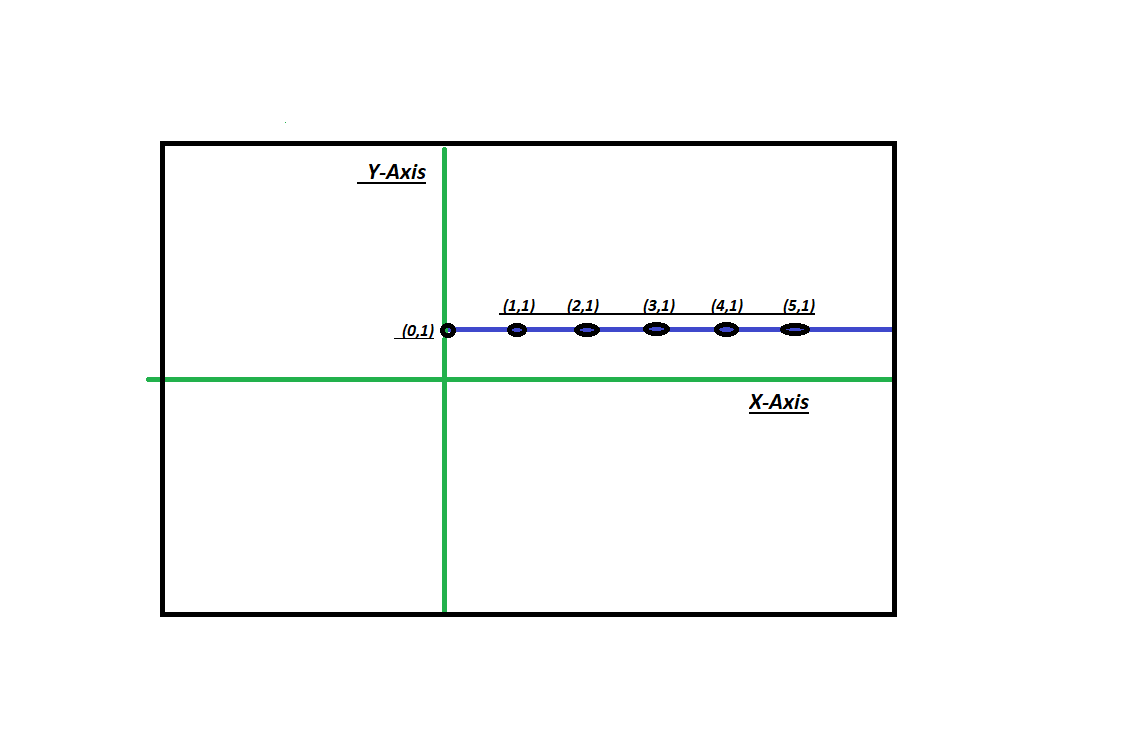# Program to check if the points are parallel to X axis or Y axis

Given n points, we need to check if these n points are parallel to X axis or Y axis or to No axis.

Examples:

```Input : x[] = {0, 0, 0, 0, 0|
y[] = {9, 2, 1, 3, 4}
Output : Parallel to Y Axis

Input : x[] = {1, 2, 3|
y[] = {9, 2, 1}
Output : Not Parallel to X or Y Axis
```

## Approach

To find the points parallel to X or Y axis just check if the points are same for any axis or not. If all the points on X axis are same then the line is parallel to Y axis. If all the points on Y axis are same so the line is parallel to X axis. If none of the cases is true, then it is not parallel to any axis.
Input the value N. And then Input the value of points in a## C++

 `// CPP program to check for parallel ` `// to X and Y Axis ` `#include ` `using` `namespace` `std; ` ` `  `// To check for parallel line ` `void` `parallel(``int` `n, ``int` `a[]) ` `{ ` `    ``bool` `x = ``true``, y = ``true``; ` ` `  `    ``// checking for parallel to X and Y ` `    ``// axis condition ` `    ``for` `(``int` `i = 0; i < n - 1; i++) { ` `        ``if` `(a[i] != a[i + 1]) ` `            ``x = ``false``; ` `        ``if` `(a[i] != a[i + 1]) ` `            ``y = ``false``; ` `    ``} ` ` `  `    ``// To display the output ` `    ``if` `(x) ` `        ``cout << ``"parallel to Y Axis"` `<< endl; ` `    ``else` `if` `(y) ` `        ``cout << ``"parallel to X Axis"` `<< endl; ` `    ``else` `        ``cout << ``"Not parallel to X"` `             ``<< ``" and Y Axis"` `<< endl; ` `} ` ` `  `// Driver's Code ` `int` `main() ` `{ ` `    ``int` `a[] = { { 1, 2 }, ` `                   ``{ 1, 4 }, ` `                   ``{ 1, 6 }, ` `                   ``{ 1, 0 } }; ` ` `  `    ``int` `n = ``sizeof``(a) / ``sizeof``(a); ` `    ``parallel(n, a); ` `    ``return` `0; ` `} `

## Java

 `// Java program to illustrate.. ` `// To check for parallel ` `// To X and Y Axis ` ` `  `import` `java.io.*; ` `import` `java.util.*; ` ` `  `class` `GFG { ` ` `  `    ``// To check for parallel line ` `    ``static` `void` `parallel(``int` `a[][]) ` `    ``{ ` `        ``boolean` `x = ``true``, y = ``true``; ` ` `  `        ``// checking for parallel to X and Y ` `        ``// axis condition ` `        ``for` `(``int` `i = ``0``; i < a.length - ``1``; i++) { ` `            ``if` `(a[i][``0``] != a[i + ``1``][``0``]) ` `                ``x = ``false``; ` `            ``if` `(a[i][``1``] != a[i + ``1``][``1``]) ` `                ``y = ``false``; ` `        ``} ` ` `  `        ``// To display the output ` `        ``if` `(x) ` `            ``System.out.println(``"Parallel to Y Axis"``); ` `        ``else` `if` `(y) ` `            ``System.out.println(``"Parallel to X Axis"``); ` `        ``else` `            ``System.out.println(``"Not parallel to X"` `                               ``+ ``" and Y axis"``); ` `    ``} ` ` `  `    ``public` `static` `void` `main(String[] args) ` `    ``{ ` `        ``int` `a[][] = { { ``1``, ``2` `}, ` `                      ``{ ``1``, ``4` `}, ` `                      ``{ ``1``, ``6` `}, ` `                      ``{ ``1``, ``0` `} }; ` `        ``parallel(a); ` `    ``} ` `} `

## Python3

 `# Python3 program to check for parallel ` `# to X and Y Axis ` ` `  `# To check for parallel line ` `def` `parallel(n, a): ` `    ``x ``=` `True``;  ` `    ``y ``=` `True``; ` ` `  `    ``# checking for parallel ` `    ``# to X and Y axis condition ` `    ``for` `i ``in` `range``(n ``-` `1``): ` `            ``if` `(a[i][``0``] !``=` `a[i ``+` `1``][``0``]): ` `                ``x ``=` `False``; ` `            ``if` `(a[i][``1``] !``=` `a[i ``+` `1``][``1``]): ` `                ``y ``=` `False``; ` ` `  `    ``# To display the output ` `    ``if` `(x): ` `        ``print``(``"Parallel to Y Axis"``); ` `    ``elif` `(y): ` `        ``print``(``"Parallel to X Axis"``); ` `    ``else``: ` `        ``print``(``"Not Parallel to X and Y Axis"``); ` ` `  `# Driver's Code ` `a ``=` `[[``1``, ``2``], [``1``, ``4``],  ` `     ``[``1``, ``6``], [``1``, ``0``]]; ` ` `  `n ``=` `len``(a); ` `parallel(n, a); ` ` `  `# This code is contributed by mits `

## C#

 `// C# program to illustrate.. ` `// To check for parallel ` `// To X and Y Axis ` ` `  `class` `GFG { ` ` `  `    ``// To check for parallel line ` `    ``static` `void` `parallel(``int``[, ] a) ` `    ``{ ` `        ``bool` `x = ``true``, y = ``true``; ` ` `  `        ``// checking for parallel to X and Y ` `        ``// axis condition ` `        ``for` `(``int` `i = 0; i < a.Rank - 1; i++) { ` `            ``if` `(a[i, 0] != a[i + 1, 0]) ` `                ``x = ``false``; ` `            ``if` `(a[i, 1] != a[i + 1, 1]) ` `                ``y = ``false``; ` `        ``} ` ` `  `        ``// To display the output ` `        ``if` `(x) ` `            ``System.Console.WriteLine(``"Parallel to Y Axis"``); ` `        ``else` `if` `(y) ` `            ``System.Console.WriteLine(``"Parallel to X Axis"``); ` `        ``else` `            ``System.Console.WriteLine(``"Not parallel to X"` `                                     ``+ ``" and Y axis"``); ` `    ``} ` ` `  `    ``public` `static` `void` `Main() ` `    ``{ ` `        ``int``[, ] a = { { 1, 2 }, ` `                      ``{ 1, 4 }, ` `                      ``{ 1, 6 }, ` `                      ``{ 1, 0 } }; ` `        ``parallel(a); ` `    ``} ` `} ` `// This code is contributed by mits `

## PHP

 ` `

Output:

```Parallel to Y Axis
```

GeeksforGeeks has prepared a complete interview preparation course with premium videos, theory, practice problems, TA support and many more features. Please refer Placement 100 for details

My Personal Notes arrow_drop_upCheck out this Author's contributed articles.

If you like GeeksforGeeks and would like to contribute, you can also write an article using contribute.geeksforgeeks.org or mail your article to contribute@geeksforgeeks.org. See your article appearing on the GeeksforGeeks main page and help other Geeks.

Please Improve this article if you find anything incorrect by clicking on the "Improve Article" button below.